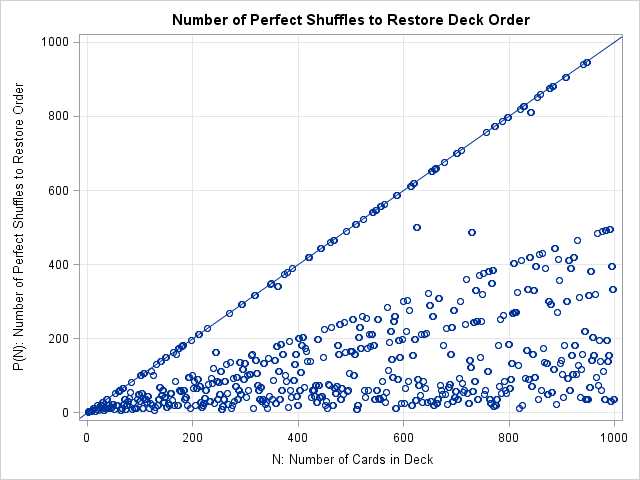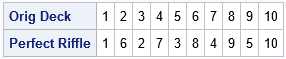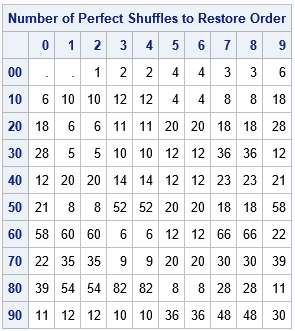Last week I compared the overhand shuffle to the riffle shuffle. I used random operations to simulate both kinds of shuffles and then compared how well they mix cards. The article caused one my colleague and fellow blogger, Rob Pratt, to ask if I was familiar with a bit of shuffling trivia: if you perform a perfect riffle shuffle, the cards return to their original order after exactly eight perfect shuffles! This mathematical curiosity is illustrated in a beautiful animation by James Miles, which shows the results of eight perfect shuffles.

After being reminded of this interesting fact, I wondered how the result generalizes to decks of various sizes. That is, if you use a deck with N cards, what is the minimum number of perfect riffle shuffles (call it P(N)) that you need to restore the deck to its original order? I decided to run a SAS program to discover the answer. The result is summarized in the following graph, which plots P(N) versus the number of cards in a deck. All points are below the identity line, which implies that at most N – 1 shuffles are required for a deck that contains N cards. If you want to learn more about the graph and its interesting patterns, read on.### Coding the perfect riffle shuffle in SAS

The perfect riffle shuffle is easier to program than the Gilbert-Shannon-Reeds (GSR) model, which uses randomness to model how real people shuffle real cards. In a perfect riffle shuffle, you cut the deck exactly two equal parts. Then you interlace the cards from the top stack with the cards from the bottom stack. The new deck ordering is TBTBTB..., where T indicates a card from the top half of the original deck and B indicates a card from the bottom half.

There are actually two perfect riffle shuffles for an even number of cards: you can interlace the cards TBTBTB..., which is called an "out shuffle," or you can interlace the cards BTBTBT..., which is called an "in shuffle." For odd-numbered decks, there is also a choice of where to cut the deck: does the top part of the deck get the extra card or does the bottom part? My program always uses the "out shuffle" convention (the top card stays on top) and gives the top stack the extra card when there is an odd number of cards. Therefore, the shuffled deck looks like TBTB...TB for even-numbered decks and TBTB...TBT for odd-numbered decks. The following SAS/IML function takes a row vector that represents a card deck and performs a perfect riffle shuffle to reorder the cards in the deck:

```proc iml; start PerfectRiffle(deck); N = ncol(deck); /* send in deck as a row vector */ shuffle = j(1,N,.); /* allocate new deck */ nT = ceil(N/2); /* cut into two stacks; "Top" gets extra card when N odd */ nB = N - nT; /* nT = size of Top; nB = size of Bottom */ shuffle[, do(1,N,2)] = deck[,1:nT]; /* top cards go into odd positions */ shuffle[, do(2,N,2)] = deck[,nT+1:N]; /* bottom cards go into even positions */ return(shuffle); finish;   /* test the algorithm on a deck that has N = 10 cards */ origDeck = 1:10; deck = PerfectRiffle(origDeck); print (origdeck//deck)[r={"Orig Deck" "Perfect Riffle"}];```The output shows one perfect riffle of a deck that has 10 cards that are originally in the order 1, 2, ..., 10.

### How many perfect riffle shuffles to restore order?

If you call the PerfectSuffle function repeatedly on the same deck, you can simulate perfect riffle shuffles. After each shuffle, you can test whether the order of the deck is in the original order. If so, you can stop shuffling. If not, you can shuffle again. The following SAS/IML loops test decks that contain up to 1,000 cards. The array nUntilRestore keeps track of how many perfect riffle shuffles were done for each deck size.

```decksize = 1:1000; /* N = 1, 2, 3, ..., 1000 */ nUntilRestore = j(1, ncol(decksize), .); /* the results vector */ do k = 2 to ncol(decksize); /* for the deck of size N */ origDeck = 1:decksize[k]; /* original order is 1:N */ deck = OrigDeck; /* set order of deck */ origOrder = 0; /* flag variable */ do nShuffles = 1 to decksize[k] until (origOrder); /* repeat shuffling */ deck = PerfectRiffle( deck ); /* shuffle deck */ origOrder = all(deck = origDeck); /* until the order of the cards are restored */ end; nUntilRestore[k] = nShuffles; /* store the number of shuffles */ end;   /* print the results for N=1,..,99 */ x = j(10, 10, .); x[2:100] = nUntilRestore[1:99]; /* present in 10x10 array */ rr = putn( do(0, 90, 10), "Z2."); /* each row has 10 elements */ cc = ("0":"9"); print x[r=rr c=cc L="Number of Perfect Shuffles to Restore Order"];   title "Number of Perfect Shuffles to Restore Deck Order"; call scatter(deckSize, nUntilRestore) grid={x y} other="lineparm x=0 y=0 slope=1;" label={"N: Number of Cards in Deck" "P(N): Number of Perfect Shuffles to Restore Order"} procopt="noautolegend";```The table shows the number of perfect riffle shuffles required for decks that have fewer than 99 cards. The results are in a 10x10 grid. The first row shows decks that have less than 10 cards, the second row shows sizes 10-19, and so on. For example, to find the result for a 52-card deck, move down to the row labeled "50" and over to the column labeled "2". The result in that cell is 8, which is the number of perfect shuffles required to restore a 52-card deck to its original order.

### Remarks on the number of perfect riffle shuffles

The graph at the top of this article shows the number of shuffles for decks that contain up to 1,000 cards. To me, the most surprising feature of the graph is the number of points that fall near diagonal lines of various rational slopes. The most apparent diagonal features are the lines whose slopes are approximately equal to 1, 1/2, and 1/3.

A complete mathematical description of this problem is beyond the scope of this blog article, but here are a few hints and links to whet your appetite.

• The integer sequence P(N) is related to the "multiplicative order of 2 mod 2n+1" in the On-Line Encyclopedia of Integer Sequences (OEIS). The encyclopedia includes a graph that is similar to the one here, but is computed for N ≤ 10,000. That integer sequence applies to the number of perfect riffle shuffles of an EVEN number of cards.
• The link to the OEIS shows a quick way to explicitly find P(N) for even values of N. P(N) is the smallest value of k for which 2k is congruent to 1 (mod N–1). For example, 8 is the smallest number for which 28 is congruent to 1 (mod 51) as you can compute explicitly: the value of mod(2**8, 51) is 1.
• The points in the graph for which P(N) = N-1 are all prime numbers: 2, 3, 5, 11, 13, 19, 29, 37, 53, 59, 61, 67, .... However, notice that not all prime numbers are on this list.

ShareDistinguished Researcher in Computational Statistics

Rick Wicklin, PhD, is a distinguished researcher in computational statistics at SAS and is a principal developer of PROC IML and SAS/IML Studio. His areas of expertise include computational statistics, simulation, statistical graphics, and modern methods in statistical data analysis. Rick is author of the books Statistical Programming with SAS/IML Software and Simulating Data with SAS.

1.hello Dr Wicklin,

I wonder is it possible to implement riffle w.out additional memory, aka not using
shuffle = j(1,N,.); /* allocate new deck */

thanks
alx

•You need *some* additional memory because you are overwriting the positions in the deck. You can get away with allocating half the deck to hold the top (or bottom) cars. You can also reuse the deck storage, if you don't mind losing the original order of the cards.

```start PR(deck); /* upon exit, deck contains new order; orig order is gone */ N = ncol(deck); /* send in deck as a row vector */ nT = ceil(N/2); /* cut into two stacks; "Top" gets extra card when N odd */ nB = N - nT; /* nT = size of Top; nB = size of Bottom */ bot = deck[,nT+1:N]; /* store bottom cards in temporary */ deck[, do(1,N,2)] = deck[,1:nT]; /* top cards go into odd positions */ deck[, do(2,N,2)] = bot; /* bottom cards go into even positions */ return(deck); finish;```
•thank you!